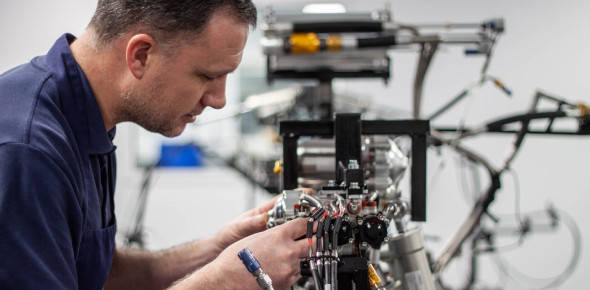# The Ultimate Technician Test! Trivia Quiz

17 Questions | Total Attempts: 148Settings• 1.
Loads are connected to a voltage source by [ ? ].
• A.

Batteries

• B.

Switches

• C.

Light bulbs

• D.

Conductors

• 2.
Increasing the voltage applied to a circuit will cause [ ? ].
• A.

Current to increase

• B.

Resistance to increase

• C.

Current to decrease

• D.

Resistance to decrease

• 3.
Resistance in a circuit is changed from 10 ohms to 5000 ohms. If the voltage remains the same, the current will [ ? ].
• A.

Double

• B.

Stay the same

• C.

Decrease

• D.

Stop flowing

• 4.
In Ohms Law, E stands for [ ? ].
• A.

Resistance

• B.

Voltage

• C.

Electromotive force

• D.

Both B and C are correct

• 5.
Four light bulbs are connected in a parallel circuit. When another bulb is connected in parallel with the other bulbs, [ ? ].
• A.

Circuit resistance will increase

• B.

Current draw will increase

• C.

Both A and B are correct

• D.

Neither A nor B are correct

• 6.
Which of the following items is used to measure angles?
• A.

A protractor

• B.

A lever

• C.

A tachometer

• D.

A gear

• 7.
A properly soldered connection will appear_______
• A.

Shiny

• B.

Dull

• C.

Dark

• D.

Greasy

• 8.
Which electronic component can store charge?
• A.

A transistor

• B.

A transformer

• C.

A resistor

• D.

A capacitor

• 9.
Most 12 V car batteries are made up of eight individual cells connected in series. What is the voltage of each cell in a car battery?
• A.

1.5 V

• B.

3 V

• C.

6 V

• D.

12 V

• 10.
What is the frequency of the alternating voltage and current typically used in the United States?
• A.

40 hz

• B.

50 hz

• C.

60 hz

• D.

110 hz

• 11.
What is the voltage across the load R and the current through R when the switch is open in the following circuit?
• A.

The current is 0 A; the voltage is 10 V.

• B.

The current is 2 A; the voltage is 10 V.

• C.

The current is 2 A; the voltage is 2 V.

• D.

The current is 0 A; the voltage is 0 V.

• 12.
An AA battery produces a potential difference of 1.5 V. Four AA batteries are placed in series to power a portable FM radio. What is the total voltage of the radio?
• A.

0.75 V

• B.

1.5 V

• C.

3.0 V

• D.

6.0 V

• 13.
Two 8-ohm speakers are connected in parallel to an amplifier with a 24-volt output. What is the voltage across each speaker?
• A.

6 volts

• B.

12 volts

• C.

18 volts

• D.

24 volts

• 14.
A current meter is connected to a circuit as shown. What does the current meter read?
• A.

3.0 amperes

• B.

4.5 amperes

• C.

13.5 amperes

• D.

15 amperes

• 15.
Which mechanical components are typically used between a wheel and an axle to reduce friction?
• A.

Springs

• B.

Hinges

• C.

Bearings

• D.

Levers

• 16.
A block of steel has a density of 0.29 pounds per cubic inch. If the block has dimensions of 1 inch by 1 inch by 3 inches, what is its weight?
• A.

0.29 pounds

• B.

0.58 pounds

• C.

0.87 pounds

• D.

1.0 pounds

• 17.
In the diagram shown above, the spring is very stiff and can be stretched 1 inch by a pulling force of 100 pounds. How much force must be applied to the block in order to move the box 3.5 inches to the left?
• A.

150 pounds

• B.

250 pounds

• C.

350 pounds

• D.

450 pounds

Related TopicsBack to top